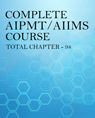×#### Thank you for registering.

One of our academic counsellors will contact you within 1 working day.

Click to Chat

1800-1023-196

+91-120-4616500

CART 0

• 0

MY CART (5)

Use Coupon: CART20 and get 20% off on all online Study Material

ITEM
DETAILS
MRP
DISCOUNT
FINAL PRICE
Total Price: Rs.

There are no items in this cart.
Continue Shopping
```
Two satellites of masses m 1 and m 2 (m 1 =9m 2 ) are revolving around the earth in circular orbits of radii r and 9r. What is the ratio of the speeds of the satellites?
Two satellites of masses m1 and m2 (m1=9m2) are revolving around the earth in circular orbits of radii r and 9r. What is the ratio of the speeds of the satellites?

```
3 years ago

```							We know that , centripetal force is F(c)=mv^2/r.Gravitational force is F(g)=GMm/r^2.Where,m=satellite massM=Earth massV=orbital velocityr=orbital radius G=gravitational constant...Since satellites are executing circular motion around Earth,so,equqting both forces,mv^2/r = GMm/r^2....,v^2=GM/r.....this is the relation between orbital radius and orbital velocity....Now for ratio of speed of satellites...v(1)^2/v(2)^2 = r(2)/r(1).....Here,,r(2)=9r,,,r(1)=r...now putting the value,,we get,,v(1)/v(2)=√9r/√r=3......So ratio of speed of satellites is 3:1..
```
3 years ago
Think You Can Provide A Better Answer ?

## Other Related Questions on AIPMT

View all Questions »### Course Features

• 731 Video Lectures
• Revision Notes
• Previous Year Papers
• Mind Map
• Study Planner
• NCERT Solutions
• Discussion Forum
• Test paper with Video Solution### Course Features

• 728 Video Lectures
• Revision Notes
• Test paper with Video Solution
• Mind Map
• Study Planner
• NCERT Solutions
• Discussion Forum
• Previous Year Exam Questions